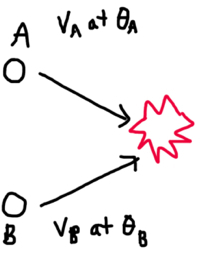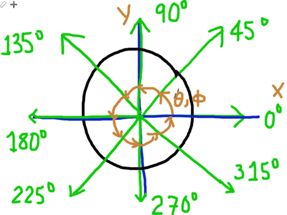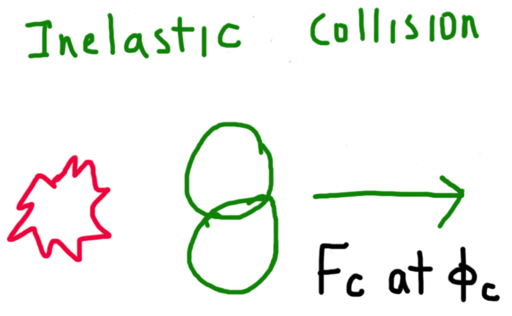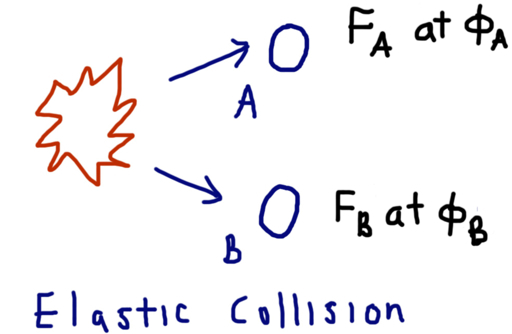## Wednesday, March 21, 2012

### Collisions: Elastic and InelasticWelcome to blog entry #65! This is the first blog entry using the new Apple iPad (3rd generation, white with 64 GB, so excited)

Introduction

In physics when two objects collide, in general, one of two things can happen:

* They can stick together, producing an inelastic collision, or

* They can bounce off of each other, producing an elastic collision.

In realty, each collision lies somewhere in between inelastic and perfectly elastic, but we will deal with the general cases here.

As the diagram above shows objects A and B are headed on a collision course; each one with their own mass, velocity, and direction. If A and B combine, let C represent the object formed from both A and B.

Define the following variables as:

MA = mass of object A
MB = mass of object B
MC = combined mass of objects A and B = mass of object C

Prior to Collision
VA = initial velocity of object A
VB = initial velocity of object B
θA = initial angle of direction of object A
θB = initial angle of direction of object B

After Collision - Inelastic Collision
FC = final velocity of the aggregate object after objects A and B collide
ΦC = final angle of the aggregate object after objects A and B collide

After Collision - Elastic Collision
FA = final velocity of object A
FB = final velocity of object B
ΦA = final angle of object A
ΦB = final angle of object B

In this blog entry, we will assume we know all the initial conditions VA, VB, θA, and θB. Additionally, assume that friction is negligible, and not a factor in calculation.

Angle is used to dictate the direction of the object. 0° is considered going due right (east) (postitive in the x-direction only). If the direction angle is 90°, the object is going due up (north), positive in the y direction only. Refer to the diagram below.Inelastic Collisions

In inelastic collisions, when objects collide, they tend to "stick" together. Examples include a car wreck or a when football (rugby) defensive end tackles a wide receiver.

Only momentum is conserved in inelastic collisions. Kinetic energy that was given by the two objects is lost. Some energy is either vibrations or heat given by the combined object.

The equation for momentum is: P = m * v, where m is mass and v is velocity.

When solving for the general case, we have to deal with the total momentum in both the x-direction and the y-direction.

The initial momentum for each direction respectively is:

Px = MA * VA * cos θA + MB * VB * cos θB (1)
Py = MA* VA *sin θA + MB * VB * sin θB (2)

Since momentum is conserved, the total initial momentum must be equal to the total final momentum, in the x- and y-direction. Hence:

Px = MC * FC * cos ΦC (3)
Py = MC * FC * sin ΦC (4)

where MC = MA + MB (5)

As a result, we have two equations of two unknowns: VC and ΦC. A calculator (such as HP 50G, TI 89, TI nSpire) or a sufficient mathematics computer program can solve the set of equations easily.

Since the equations are relatively simple, we can find explicit solutions by the following strategy.

First, solve for ΦC. Either equation (3) or (4) can be used. For this blog entry, I will use equation (3).

Px = MC * FC * cos ΦC
Px / (MC * FC) = cos ΦC

Then ΦC = arccos (Px / (MC * FC)) (6)

Sub into (4) to get:

Py = MC * FC * sin (arccos (Px / (MC * FC)))

Note that sin(arccos x) = √ (1 - x^2). Also cos(arcsin x) = √ (1 - x^2)

Then:

Py = MC * FC * √ ( 1 - (Px / (MC * FC))^2 )
Py = MC * FC * √ ( ( MC^2 FC^2 - Px^2 ) / (MC^2 * FC^2) )
Py = (MC * FC * √ ( MC^2 FC^2 - Px^2 ) ) / (MC * FC)
Py = √ ( MC^2 * FC^2 - Px^2 )

Square both sides and rearrange terms:

MC^2 * FC^2 = Py^2 + Px^2

Solving for FC gives:

FC = √ ( (Py^2 + Px^2) / MC^2 ) (7)

(We can just take the principal root, since the ΦC will take care of the direction of object C)

In Summary:
MC = MA + MB
FC = √ ( (Py^2 + Px^2) / MC^2 )
ΦC = arccos (Px / (MC * FC))

This is a solution for an inelastic collision for any two objects of any mass, velocity, and direction angle.Elastic Collisions

In perfectly elastic collisions, when objects collide, they tend to "bounce off" each other. Examples include a sub-atomic gas particle collision or two billiard balls hitting each other.

Like inelastic collisions, momentum is conserved in inelastic collisions. Unlike inelastic collisions, kinetic energy is also conserved.

The equation for momentum is: P = m * v, where m is mass and v is velocity.

When solving for the general case, we have to deal with the total momentum in both the x-direction and the y-direction.

The initial momentum for each direction respectively is:

Px = MA * VA * cos θA + MB * VB * cos θB (1)
Py = MA* VA *sin θA + MB * VB * sin θB (2)

The equation for kinetic energy is: KE = 1/2 * m * v^2. Kinetic energy does not take separate compenent directions into account.

The initial kinetic energy is:

KE = 1/2 * MA * VA^2 + 1/2 * MB * VB^2 (8)

Since momentum and kinetic energy are conserved, the total initial momentum must be equal to the total final momentum, in the x- and y-direction; and the total initial kinetic energy must be equal to the total final kinetic energy. Hence:

Px = MA * FA * cos ΦA + MB * FB * cos ΦB (9)
Py = MA * FA * sin ΦA + MB * FB * sin ΦB (10)
KE = 1/2 * MA * FA^2 + 1/2 * MB * FB^2 (11)

Note if we do not know anything about the final conditions, then we are dealing with a system of three equations with four unknowns: FA, ΦA, FB, and ΦB.

In order for this system to be solvable, we must know one of the final conditions. Then the system becomes three equations and three unknowns. A calculator (such as HP 50G, TI 89, TI nSpire) or mathematics computer program can assist in solving the set of equations.

What if one of the velocities is known?

Let's assume that FA is known. We can simplify the system of equations (9), (10), and (11). A similar strategy can be used if FB is known.

KE = 1/2 * MA * FA^2 + 1/2 * MB * FB^2
1/2 * MB * FB^2 = KE - 1/2 * MA * FA^2
FB^2 = 2 * KE / MB - MA * FA^2 / MB

Then:

FB = √ ( ( 2 * KE - MA * FA^2 ) / MB ) (12)

Now we are left with the system of two equations with two unknowns: ΦA and ΦB. Use your favorite calculating tool to solve for them.

Px = MA * FA * cos ΦA + MB * FB * cos ΦB (9)
Py = MA * FA * sin ΦA + MB * FB * sin ΦB (10)

For more information of elastic and inelastic collisions, click here (Hyperphysics Page). . Thanks Mime Grigsby, a very good friend of mine and fellow mathematician.

Until next time,

Eddie

Diagrams: drawn using Whiteboard

This blog entry is dedicated to Uncle Ralph (born March 21). Me and my family miss you and love you. Thank you for touching our lives.

This blog entry is property of Edward Shore. © 2012

### Retro Review: Radio Shack EC-4026

Retro Review:   Radio Shack EC-4026 Quick Facts: Model:  EC-4026 Company:  Radio Shack Equivalent Of:  Casio fx-4500P, Casio fx-4500PA Years...